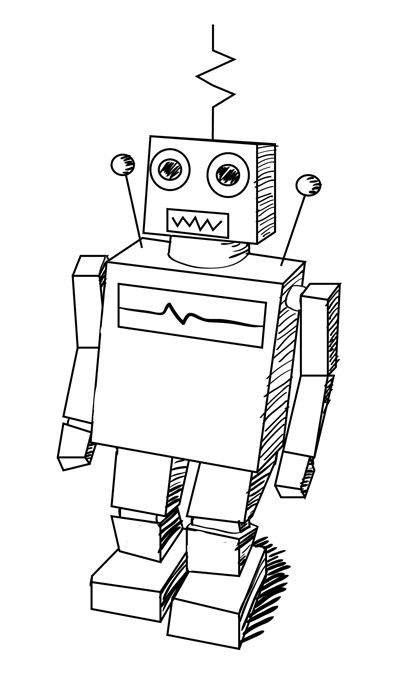# Classification Versus Regression — Intro To Machine Learning #5

Often when a machine learning task is presented to you the first thing you will do it’s to get to know whether the learning task is Classification or regression problem so that next you can pick the algorithm. These are simple concepts to understand, watch out.

# Classification

In classification problems we are trying to predict a discrete number of values.

The labels(y) generally comes in categorical form and represents a finite number of classes. Consider the tasks bellow:

1. Given set of input features predict whether a Breast Cancer is Benign or Malignant.
2. Given an image correctly classify as containing Cats or Dogs.
3. From a given email predict whether it’s spam email or not.

## Types of classification

(1). Binary classification — when there is only two classes to predict, usually 1 or 0 values.

Multi-Class Classification — When there are more than two class labels to predict we call multi-classification task. E.g. predicting 3 types of iris species, image classification problems where there are more than thousands classes(cat, dog, fish, car,…).

## Algorithms for classification

• Decision Trees
• Logistic Regression
• Naive Bayes
• K Nearest Neighbors
• Linear SVC (Support vector Classifier)
• etc

# Regression Problems

In regression problems we trying to predict continuous valued output, take this example. Given a size of the house predict the price(real value).

## Regression Algorithms

• Linear Regression
• Regression Trees(e.g. Random Forest)
• Support Vector Regression (SVR)
• etc

# Classification VS Regression

Classification: Discrete valued Y (e.g. 1,2,3 and 4)

Regression: Continues Values Y (e.g. 222.6, 300, 568,…)

Whenever you find machine learning problem first define whether you are dealing with a classification or regression problem and you can get to know that analyzing the target variable (Y), note that here the input X can of any kind (continues or discrete) that doesn’t count to define the problem. After defining the problem and getting to know the data it’s much easier to chose or try out some algorithms.

# There is More

Besides classification and regression actually there’s also something called clustering. In clustering the primary objective is to create groups (clusters) based on the similarities of the examples (We’ll come to this topic later).

Check this out:

## Next:

In the next article we are going to talk about Linear Regression Algorithm.

Please let me know what you think by clicking ‘like’ or leave me a comment

Happy learning.

Written by

Written by

## David Fumo

#### love coding, read, write and create. Interested in AI, Blockchain, Startups, Growth and Entrepreneurship.## More From Medium

Welcome to a place where words matter. On Medium, smart voices and original ideas take center stage - with no ads in sight. Watch
Follow all the topics you care about, and we’ll deliver the best stories for you to your homepage and inbox. Explore
Get unlimited access to the best stories on Medium — and support writers while you’re at it. Just \$5/month. Upgrade